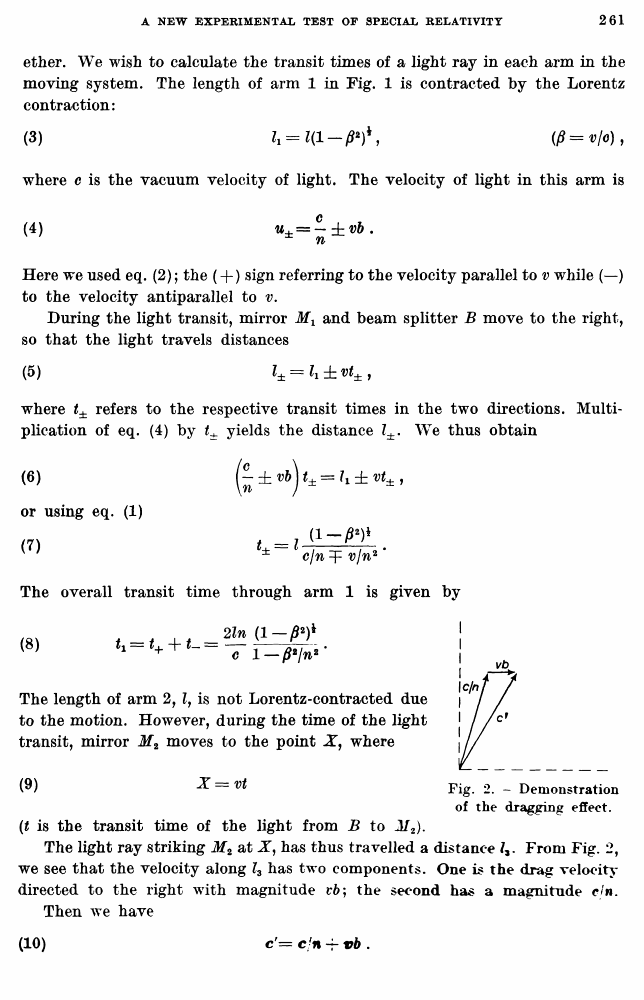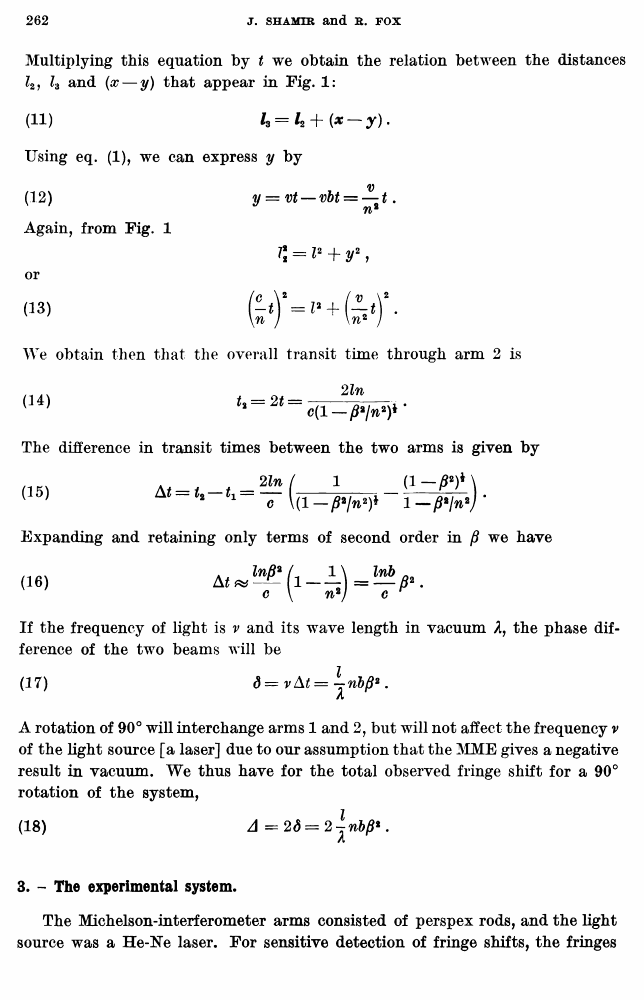Shamir, J.; Fox, R. A new experimental test of special relativity // Nuovo Cimento B Series 10, vol. 62, issue 2, 11 Aug. 1969

В начало   Другие форматы   <<<     Страница 261   >>>258  259  260  261 262  263  264

ether. We wish to calculate the transit times of a light ray in each arm in the moving system. The length of arm 1 in Fig. 1 is contracted by the Lorentz contraction:

where c is the vacuum velocity of light. The velocity of light in this arm is

Here we used eq. (2); the (+ ) sign referring to the velocity parallel to v while (—) to the velocity antiparallel to v.

During the light transit, mirror Mx and beam splitter B move to the right, so that the light travels distances

where t± refers to the respective transit times in the two directions. Multiplication of eq. (4) by t± yields the distance Z±. We thus obtain

(t is the transit time of the light from B to M2).

The light ray striking M2 at X, has thus travelled a distance From Fig. 2, we see that the velocity along l3 has two components. One is the drag velocity directed to the right with magnitude t*6; the second has a magnitude cjn. Then we have

W

(6)

or using eq. (1) (7)

The overall transit time through arm 1 is given by

(8)

The length of arm 2, lf is not Lorentz-contracted due to the motion. However, during the time of the light transit, mirror M2 moves to the point X, where

(9)

X=vt

Fig. 2. - Demonstration of the dragging effect.

(10)

c'= c/n vb .

Multiplying this equation by t we obtain the relation between the distances Z2, h and (x — y) that appear in Fig. 1:

(11)

Using eq. (1), we can express y by (12)

Again, from Fig. 1

or

(13)

We obtain then that the overall transit time through arm 2 is

(14)

The difference in transit times between the two arms is given by

(15)

Expanding and retaining only terms of second order in /? we have

(16)

If the frequency of light is v and its wave length in vacuum A, the phase difference of the two beams will be

(17)

A rotation of 90° will interchange arms 1 and 2, but will not affect the frequency v of the light source [a laser] due to our assumption that the MME gives a negative result in vacuum. We thus have for the total observed fringe shift for a 90° rotation of the system,

(IB)
3. - The experimental system.

The Michelson-interferometer arms consisted of perspex rods, and the light source was a He-Ne laser. For sensitive detection of fringe shifts, the fringes